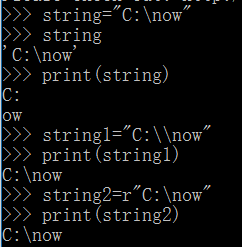Mike学python（1）：python的基础知识

下面直接贴出一段用于猜数的python简单代码，通过这段代码来解析python编程的一些特点

import random
secret=random.randint(1,9)
temp=input(“input a number”)
guess=int(temp)
while(secret!=guess):
if(secret>guess):
print(“input a bigger number”)
else:
print(“input a smaller number”)
temp=input(“input a number again”)
guess=int(temp)
print(“bingo”)

5.print(string)打印字符串时可能会出现误认为为转移字符的情况，其两种解决方案也如下：（r:raw）算术运算符

/ :精确除法（10/4=2.5）
// :地板除法（10//4=2）
** :幂运算（3**2=9）

3<4<5 是合法的表达式